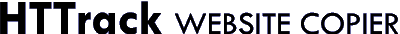Author: Anders
Date: 07/14/2015 08:13
 Hi, Im downloading a math page, and its all working fine except for the expressions writting in some non-html thing -(i think). For example a/sin(A) = b/sin(B) = c/sin(C) turns into: $$\frac{a}{\sin(A)}=\frac{b}{\sin(B)}=\frac{c}{\sin(C)}$$ When ripped. Is that fixable? Regards

All articles